# 7. Cosmology 1 Is the Universe Closed?

 we can determine the metric on the hyperboloid surface by substituting the squared differentials (dT)2 and (dW)2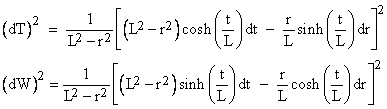into the five-dimensional metric, which gives equation (1). The accelerating expansion of the space for a positive cosmological constant can be regarded as a consequence of a universal repulsive force. The radius of the spatial sphere follows a hyperbolic trajectory similar to the worldlines of constant proper acceleration discussed in Section 2.9. To show that the expansion of the de Sitter spacetime can be seen as exponential, we can put the metric into the “Robertson-Walker form” (see Section 7.1) by defining a new system of coordinates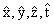such that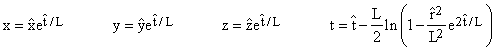where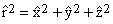It follows that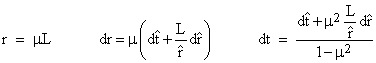where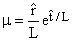Substituting into the metric (1) gives the exponential form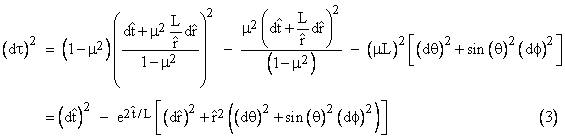This the characteristic length R(t) for this metric is the simple exponential function. (This form of the metric covers only part of the manifold.) Equations (1), (2), and (3) are the most common ways of expressing de Sitter’s metric, but in the first letter that de Sitter wrote to Einstein on this subject he didn’t give the line element in any of these familiar forms. We can derive his original formulation beginning with (1) if we define new coordinates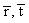related to the r,t coordinates of (1) by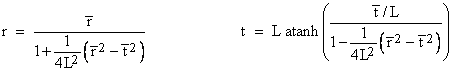Incidentally, the t coordinate is the “relativistic difference” between the advanced and retarded combinations of the barred coordinates, i.e.,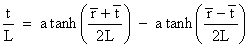The differentials in (1) can be expressed in terms of the barred coordinates as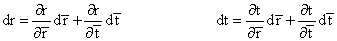where the partials are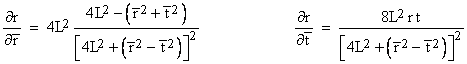and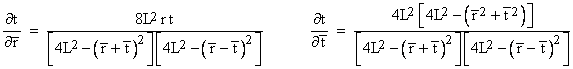Making these substitutions and simplifying, we get the “Cartesian” form of the metric that de Sitter presented in his first letter to Einstein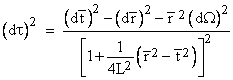where d denotes the angular components, which are unchanged from (1). These expressions have some purely mathematical features of interest. For example, the line element is formally similar to the expressions for curvature discussed in Section 5.3. Also, the denominators of the partials of t are, according to Heron’s formula, equal to 16A2 where A is the area of a triangle with edge lengths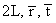.   If the cosmological constant was zero (meaning that L was infinite) all the dynamic solutions of the field equations with matter predict a slowing rate of expansion, but in 1998 two independent groups of astronomers reported evidence that the expansion of the universe is actually accelerating. If these findings are correct, then some sort of repulsive force is needed in models based on general relativity. This has led to renewed interest in the cosmological constant and de Sitter spacetime, which is sometimes denoted as dS4. If the cosmological constant is negative the resulting spacetime manifold is called anti-de Sitter spacetime, denoted by AdS4. In the latter case, we still get a hyperboloid, but the time coordinate advances circumferentially around the surface. To avoid closed time-like curves, we can simply imagine “wrapping” sheets around the hyperboloid.   As discussed in Section 7.1, the characteristic length R(t) of a manifold (i.e., the time-dependent coefficient of the spatial part of the manifold) satisfying the modified Einstein field equations (with non-zero cosmological constant) varies as a function of time in accord with the Friedmann equation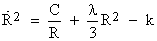where dots signify derivatives with respect to a suitable time coordinate, C is a constant, and k is the curvature index, equal to either -1, 0, or +1. The terms on the right hand side are akin to potentials, and it’s interesting to note that the first two terms correspond to the two hypothetical forms of gravitation highlighted by Newton in the Principia. (See Section 8.2 for more on this.) As explained in Section 7.1, the Friedmann equation implies that R satisfies the equation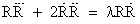which shows that, if  = 0, the characteristic cosmological length R is a solution of the “separation equation” for non-rotating gravitationally governed distances, as given by equation (2) of Section 4.2. Comparing the more general gravitational separation from Section 4.2 with the general cosmological separation, we have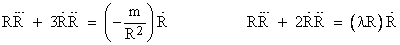which again highlights the inverse square and the direct proportionalities that caught Newton’s attention. It’s interesting that with m = 0 the left-hand expression reduces to the purely inertial separation equation, whereas with  = 0 the right hand expression reduces to the (non-rotating) gravitational separation equation. We saw that the “homogeneous” forms of these equations are just special cases of the more general relation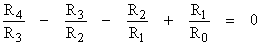where subscripts denote derivatives with respect to a suitable time coordinate. Among the solutions of this equation, in addition to the general co-inertial separations, non-rotating gravitational separations, and rotating-sliding separations, are sinusoidal functions and exponential functions. Historically this led to the suspicion, long before the recent astronomical observations, that there might be a class of exponential cosmological distances in addition to the cycloidal and parabolic distances. In other words, there could be different classes of observable distances, some very small and oscillatory, some larger and slowing, and some – the largest of all – increasing at an accelerating rate. This is illustrated in the figure below.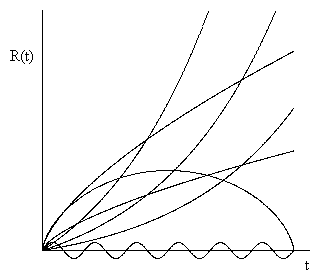Of course, according to all conventional metrical theories, including general relativity, the spatial relations between material objects (on any chosen temporal foliation) conform to a single three-dimensional manifold. Assuming homogeneity and isotropy, it follows that all the cosmological distances between objects are subject to the ordinary metrical relations such as the triangle inequality. This greatly restricts the observable distances. On the other hand, our assumption that the degrees of freedom are limited in this way is based on our experience with much smaller distances. We have no direct evidence that cosmological distances are subject to the same dependencies. As an example of how concepts based on limited experience can be misleading, recall how special relativity revealed that the metric of our local spacetime fails to satisfy the axioms of a metric, including the triangle inequality. The non-additivity of relative speeds was not anticipated based on human experience with low speeds. Likewise for three “co-linear” objects A,B,C, it’s conceivable that the distance AC is not the simple sum of the distances AB and BC. The feasibility of regarding separations (rather than particles) as the elementary objects of nature was discussed in Section 4.1.   One possible observational consequence of having distances of several different classes would be astronomical objects that are highly red-shifted and yet much closer to us than the standard Hubble model would imply based on their redshifts. (Of course, even if this view was correct, it might be the case that all the exponential separations have already passed out of view.) Another possible consequence would be that some observable distances would be increasing at an accelerating rate, whereas others of the same magnitude might be decelerating.   The above discussion shows that the idea of at least some cosmological separations increasing at an accelerating rate can (and did) arise from completely a priori considerations. Of course, as long as a single coherent expansion model is adequate to explain our observations, the standard GR models of a smooth manifold will remain viable. Less conventional notions such as those discussed above would only be called for only if we begin to see conflicting evidence, e.g., if some observations strongly indicate accelerating expansion while others strongly indicate decelerating expansion.   The cosmological constant is hardly ever discussed without mentioning that (according to Gamow) Einstein called it his “biggest blunder”, but the reasons for regarding this constant as a “blunder” are seldom discussed. Some have suggested that Einstein was annoyed at having missed the opportunity to predict the Hubble expansion, but in his own writings Einstein argued that “the introduction of [the cosmological constant] constitutes a complication of the theory, which seriously reduces its logical simplicity”. He also wrote “If there is no quasi-static world, then away with the cosmological term”, adding that it is “theoretically unsatisfactory anyway”. In modern usage the cosmological term is usually taken to characterize some feature of the vacuum state, and so it is a fore-runner of the extremely complicated vacua that are contemplated in the “string theory” research program. If Einstein considered the complication and loss of logical simplicity associated with a single constant to be theoretically unsatisfactory, he would presumably have been even more dissatisfied with the nearly infinite number of possible vacua contemplated in current string research. Oddly enough, the de Sitter and anti-de Sitter spacetimes play a prominent role in this research, especially in relation to the so-called AdS/CFT conjecture involving conformal field theory.  Download 0.57 Mb.Share with your friends: